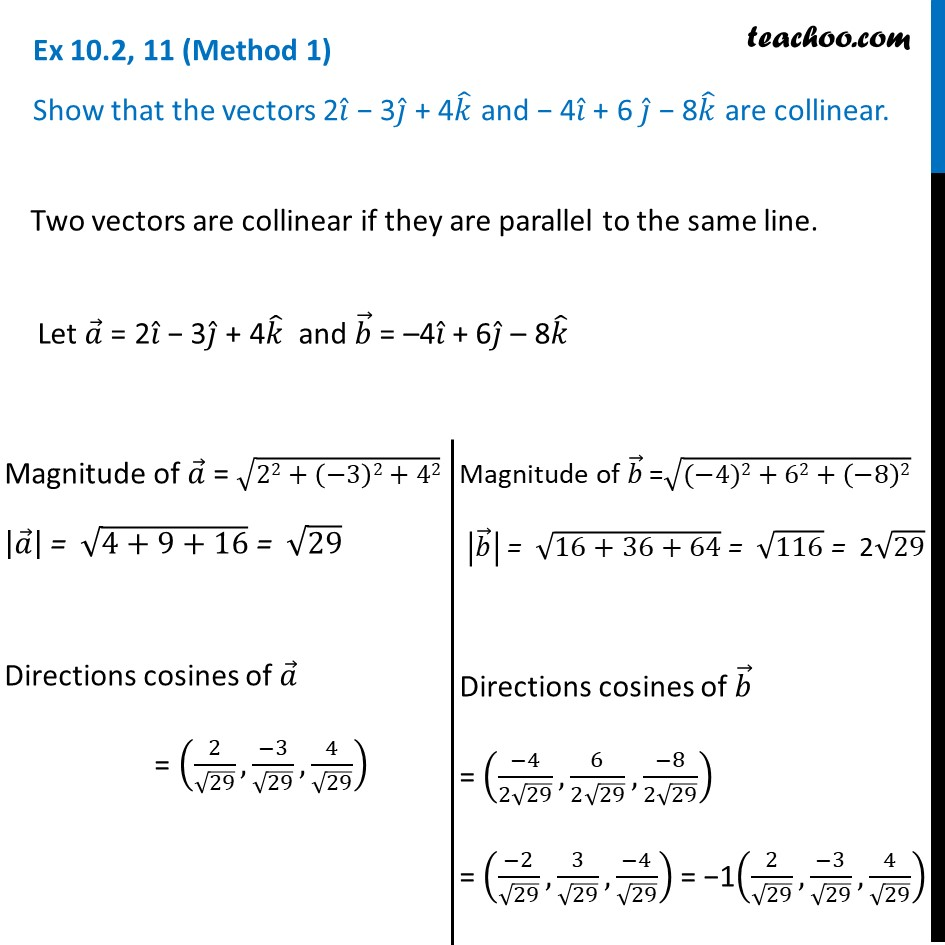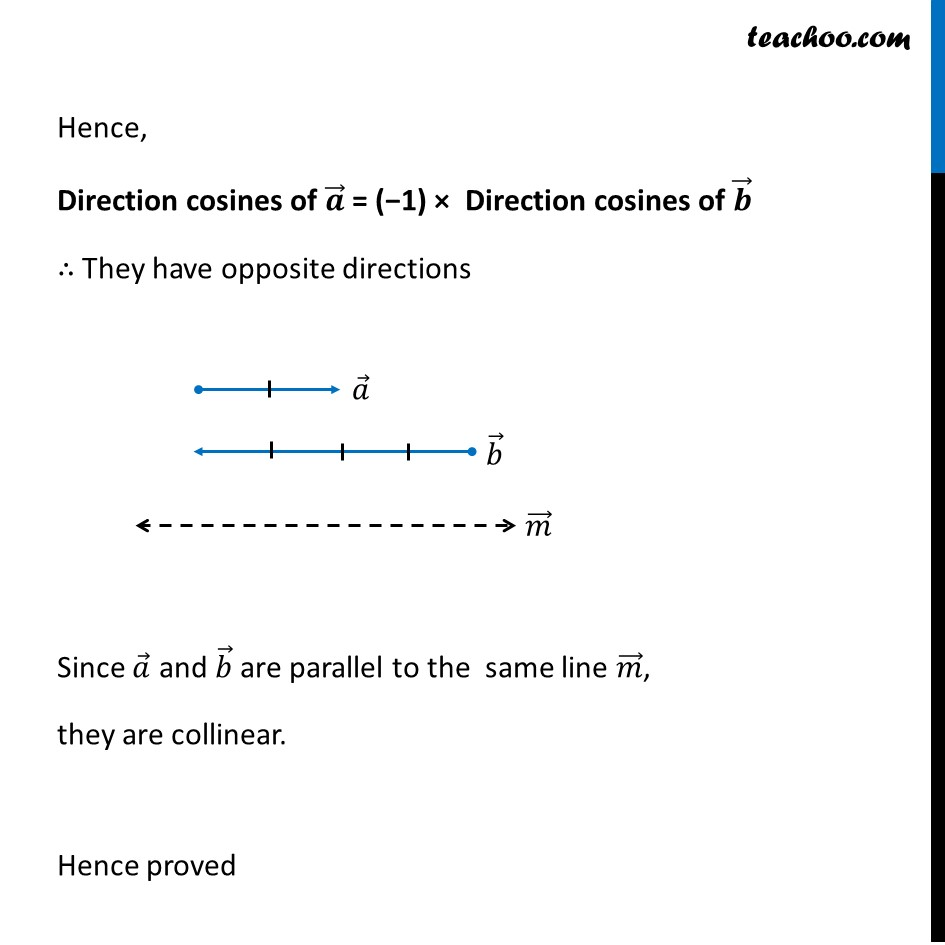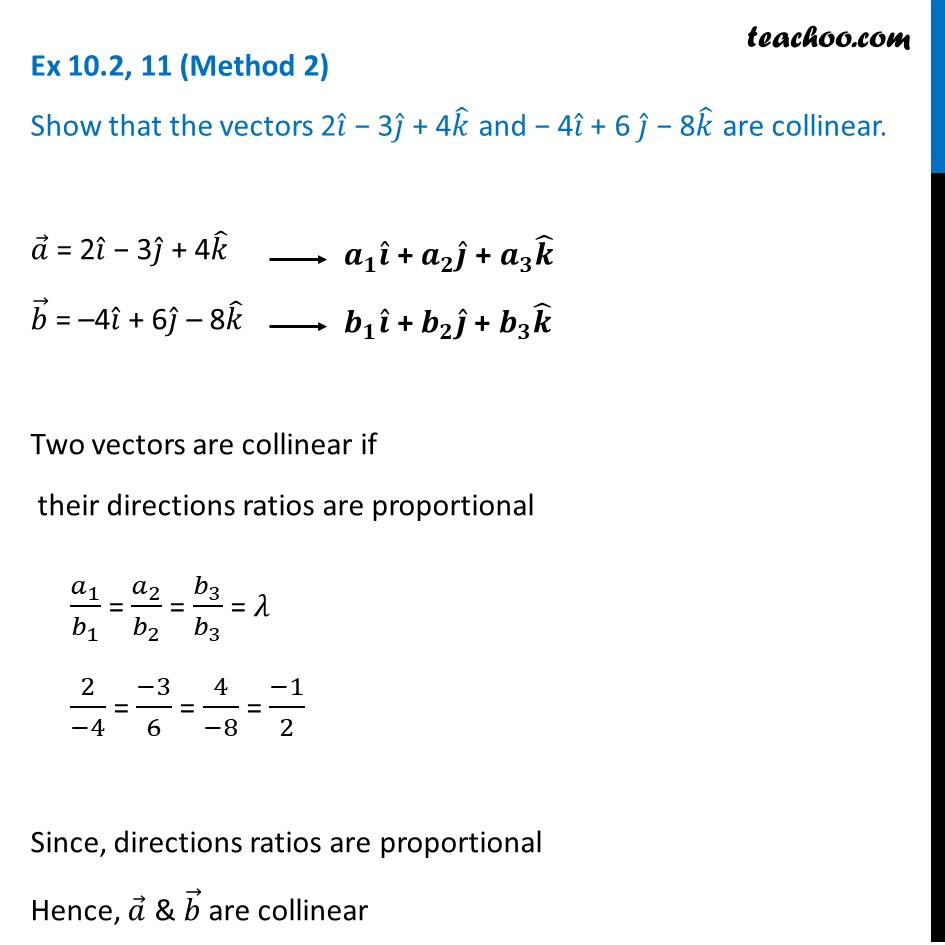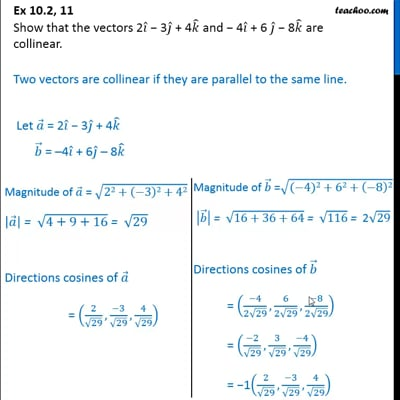Ex 10.2

Chapter 10 Class 12 Vector Algebra
Serial order wiseThis video is only available for Teachoo black users

Solve all your doubts with Teachoo Black (new monthly pack available now!)

### Transcript

Ex 10.2, 11 (Method 1) Show that the vectors 2𝑖 ̂ − 3𝑗 ̂ + 4𝑘 ̂ and − 4𝑖 ̂ + 6 𝑗 ̂ − 8𝑘 ̂ are collinear.Two vectors are collinear if they are parallel to the same line. Let 𝑎 ⃗ = 2𝑖 ̂ − 3𝑗 ̂ + 4𝑘 ̂ and 𝑏 ⃗ = –4𝑖 ̂ + 6𝑗 ̂ – 8𝑘 ̂ Magnitude of 𝑎 ⃗ = √(22+(−3)2+42) |𝑎 ⃗ | = √(4+9+16) = √29 Directions cosines of 𝑎 ⃗ = (2/√29,(−3)/√29,4/√29) Magnitude of 𝑏 ⃗ =√((−4)2+62+(−8)2) |𝑏 ⃗ | = √(16+36+64) = √116 = 2√29 Directions cosines of 𝑏 ⃗ = ((−4)/(2√29),6/(2√29),(−8)/(2√29)) = ((−2)/√29,3/√29,(−4)/√29) = −1(2/√29,(−3)/√29,4/√29) Hence, Direction cosines of 𝒂 ⃗ = (−1) × Direction cosines of 𝒃 ⃗ ∴ They have opposite directions Since 𝑎 ⃗ and 𝑏 ⃗ are parallel to the same line 𝑚 ⃗, they are collinear. Hence proved Ex 10.2, 11 (Method 2) Show that the vectors 2𝑖 ̂ − 3𝑗 ̂ + 4𝑘 ̂ and − 4𝑖 ̂ + 6 𝑗 ̂ − 8𝑘 ̂ are collinear.𝑎 ⃗ = 2𝑖 ̂ − 3𝑗 ̂ + 4𝑘 ̂ 𝑏 ⃗ = –4𝑖 ̂ + 6𝑗 ̂ – 8𝑘 ̂ Two vectors are collinear if their directions ratios are proportional 𝑎_1/𝑏_1 = 𝑎_2/𝑏_2 = 𝑏_3/𝑏_3 = 𝜆 2/(−4) = (−3)/6 = 4/(−8) = (−1)/2 Since, directions ratios are proportional Hence, 𝑎 ⃗ & 𝑏 ⃗ are collinear